# Selina Concise Mathematics Class 10 ICSE Solutions Chapter 3 Shares and Dividend Ex 3B

## Selina Concise Mathematics Class 10 ICSE Solutions Chapter 3 Shares and Dividend Ex 3B

These Solutions are part of Selina Concise Mathematics Class 10 ICSE Solutions. Here we have given Selina Concise Mathematics Class 10 ICSE Solutions Chapter 3 Shares and Dividend Ex 3B.

Other Exercises

Question 1.
A man buys 75, Rs. 100 shares paying 9 per cent dividend. He buys shares at such a price that he gets 12 per cent of his money. At what price did he buy the shares?
Solution:
No. of shares = 75
Value of each share = Rs. 100
Rate of dividend = 9%
Let the market value of each share = x
Thus. 12% of x = 9% of 100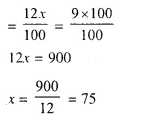Market value of each share = Rs. 75

Question 2.
By purchasing Rs. 25 gas shares for Rs. 40 each, a man gets 4 per cent profit on his investment. What rate per cent is the company paying ? What is his dividend if he buys 60 shares?
Solution:
Face value of each share = Rs. 25
Market value of each share = Rs. 40
Profit = 4% of his investment
Let the rate of dividend = x %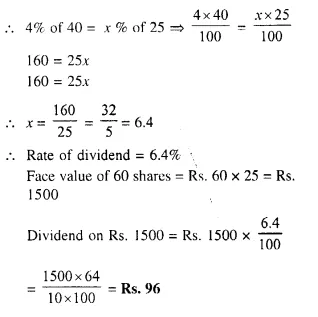Question 3.
Hundred rupee shares of a company are available in the market at a premium of Rs. 20. Find the rate of dividend given by the company, when a man’s return on his investment is 15 percent.
Solution:
Face value of each share = Rs. 100
Market value of each share = Rs. 120
Total dividend = 15% on his investment
Let the rate of dividend = x %
Then x % of 100 = 15 % of 120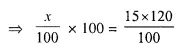x = 18%
Rate of dividend = 18%

Question 4.
Rs. 50 shares of a company are quoted at a discount of 10%. Find the rate of dividend given by the company, the return on the investment on these shares being 20 percent.
Solution:
Face value of each share = Rs. 50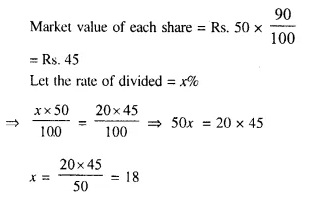Rate of dividend = 18%

Question 5.
A company declares 8 per cent dividend to the share holders. If a man receives Rs. 2,840 as his dividend, find the nominal value of his shares.
Solution:
Rate of dividend = 8%
Total dividend = Rs. 2.840
Let Nominal value of shares = x
Then 8% of x = Rs. 2840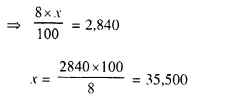Nominal value of his shares = Rs. 35,500

Question 6.
How much should a man invest in Rs. 100 shares selling at Rs. 110 to obtain an annual income of Rs. 1,680, if the dividend declared is 12% ?
Solution:
Face value of each share = Rs. 100
Market value of each share = Rs. 110
Total annual income = Rs. 1,680.
Rate of dividend = 12%
Let total amount of shares = x
Then x x 12% = 1,680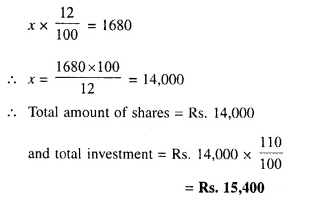Question 7.
A company declares a dividend of 11.2% to all its share-holders. If its Rs. 60 share is available in the market at a premium of 25%, how much should Rakesh invest in buying the shares of this company in order to have an annual income of Rs. 1,680 ?
Solution:
Face value of each share = Rs. 60
Market value = Rs. 60 x $$\frac { 125 }{ 100 }$$ = Rs. 75
Rate of dividend = 11.2%
Annual income = Rs. 1,680
Let the face value of shares = x
Dividend = x x 11.2%
x x 11.2% = 1680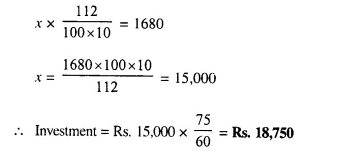Question 8.
A man buys 400, twenty rupee shares at a premium of Rs. 4 each and receives a dividend of 12%. Find :
(i) the amount invested by him.
(ii) his total income from the shares.
(iii) percentage return on his money.
Solution:
No. of shares = 400
Face value of one share = Rs. 20
Market value of one share = Rs. 20 + 4 = Rs. 24
Rate of dividend = 12%
(i) Amount invested by the man = Rs. 24 x 400 = Rs. 9600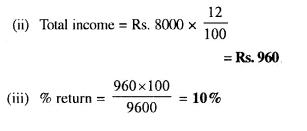Question 9.
A man buys 400, twenty-rupee shares at a discount of 20% and receives a return of 12% on his money. Calculate
(i) the amount invested by him.
(ii) the rate of dividend paid by the company.
Solution:
No. of shares = 400
Face value of one share = Rs. 20
Market value of one share = Rs. 20 x $$\frac { 80 }{ 100 }$$ = Rs. 16
Amount of shares = Rs. 20 x 400 = Rs. 8,000
(i) Amount invested = Rs. 16 x 400 = Rs. 6,400
(ii) Total dividend = Rs. 6,400 x $$\frac { 12 }{ 100 }$$ = Rs. 768
Rate of dividend = $$\frac { 768 x 100 }{ 8000 }$$= 9.6 %

Question 10.
A company with 10,000 shares of Rs. 100 each, declares an annual dividend of 5%.
(i) What is the total amount of dividend paid by the company ?
(ii) What should be the annual income of a man who has 72 shares, in the company ?
(iii) If he received only 4% of his investment, find the price he paid for each share.
Solution:
No. of shares = 10000
Face value of each share = 100
Rate of dividend = 5%
Amount of shares = Rs. 100 x 10,000 = Rs. 10,00,000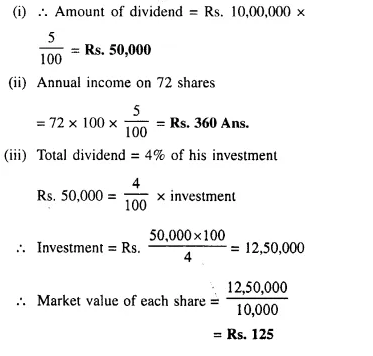Question 11.
A lady holds 1800, Rs. 100 shares of a company that pays 15 % dividend annually. Calculate her annual dividend. If she had bought these shares at 40% premium, what is the return she gets as percent on her investment. Give your answer to the nearest integer.
Solution:
No. of shares = 1800
Face value of each share = Rs. 100
Rate of dividend = 15 %
Market value of each share Rs. 140
Total value of shares = Rs. 1800 x 100 = Rs. 1,80,000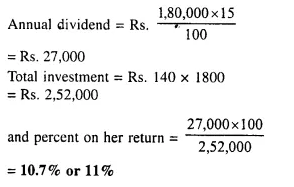Question 12.
A man invests Rs. 11,200 in a company paying 6 percent per annum when its Rs. 100 shares can be bought for Rs. 140 find:
(i) his annual dividend.
(ii) his percentage return on his investment.
Solution:
Investment = Rs. 11,200
Rate of dividend = 6%
Market value of a share = Rs. 140
No. of shares = Rs. 11,200 ÷ Rs. 140 = 80
Face value of 80 shares = 80 x Rs. 100 = Rs. 8,000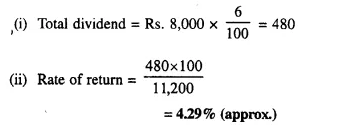Question 13.
Mr. Sharma has 60 shares of N.V. ₹ 100 and sells them when they are at a premium of 60%. He invests the proceeds in shares of nominal value ₹ 50, quoted at 4% discount, and paying 18% dividend annually. Calculate:
(i) the sale proceeds ;
(ii) the number of shares he buys and
(iii) his annual dividend from the shares.
Solution:
(i) No. of shares = 60
Face value of each share = Rs. 100
Total amount = Rs. 100 x 60 = Rs. 6,000
Market value = Rs. 160
His sale proceed = Rs. 160 x 60 = Rs. 9,600
(ii) In second case :
Nominal value of each share = Rs.50
and Market value = Rs. 50 x $$\frac { 96 }{ 100 }$$ = Rs. 48
Rate of dividend = 18%
No. of shares he purchased = $$\frac { 9600 }{ 48 }$$ = 200
(iii) Face value of 200 shares = 200 x Rs. 50 = Rs. 10,000
Dividend = Rs. 10000 x $$\frac { 18 }{ 100 }$$ = Rs. 1,800

Question 14.
A company with 10,000 shares of nominal value ₹ 100 declares an annual dividend of 8% to shareholders.
(i) Calculate the total amount of dividend paid by the company.
(ii) Ramesh had bought 90 shares of the company at ₹ 150 per share. Calculate the dividend he receives and the percentage of return on his investment.
Solution:
(i) No. of shares = 10,000
Nominal value of each share = Rs. 100
Dividend = 8%
Total face value of 10,000 shares = Rs. 100 x 10,000 = Rs. 10,00,000
and amount of dividend = Rs. $$\frac { 1000000 x 8 }{ 100 }$$ = Rs. 8000
(ii) In second case :
Ramesh bought = 90 shares
Market value of each share = Rs. 150
His investment = Rs. 150 x 90 = Rs. 13,500
Face value of 90 shares = Rs. 100 x 90 = Rs. 9,000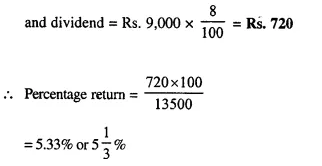Question 15.
Which is the better investment:
16% Rs. 100 shares at 80 or 20% Rs. 100 shares at 120 ?
Solution:
In first case :
Income on Rs. 80 = Rs. 16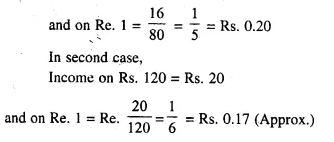From above, it is clear that first investment is better.

Question 16.
A man has a choice to invest in hundred rupee shares of two firms at Rs. 120 or at Rs. 132. The first firm pays r. dividend of 5% per annum and the second firm pays a dividend of 6% per annum. Find :
(i) Which company is giving a better return.
(ii) If a man invests Rs. 26,400 with each firm, how much will be the difference between the annual returns from the two firms.
Solution:
In first case :
Market value of share = Rs. 120
and dividend = 5%
Income on Rs. 120 = Rs. 5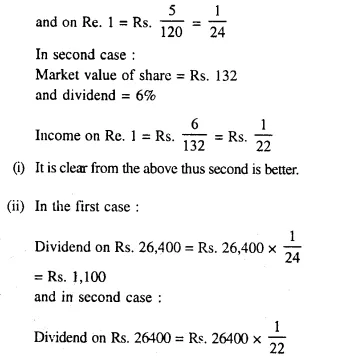= Rs. 1,200
Difference = Rs. 1,200 – Rs. 1,100 = Rs. 100

Question 17.
A man bought 360, ten rupee shares of a company paying 12 percent per annum. He sold the shares when their price rose to Rs. 21 per share and invested the proceeds in five rupee shares paying 4.5 per cent per annum at Rs 3.50 per share. Find the annual change in his income.
Solution:
No. of shares bought = 360
Face value of each share = Rs. 10
Dividend = 12%
Cost price of 360 shares = Rs. 360 x 10 = Rs. 3,600
Market value = Rs. 21
Selling price = Rs. 21 x 360 = Rs. 7,560
In second case :
Face value of each share = Rs. 5
Market value of each share = Rs. 3.5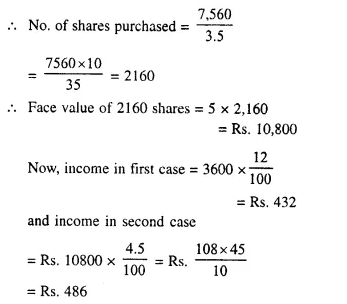Difference in his income = Rs. 486 Rs. 432 = Rs. 54

Question 18.
A man sold 400 (Rs. 20) shares of a company paying 5% at Rs. 18 and invested the proceeds in (Rs. 10) shares of another company paying 7% at Rs. 12. How many (Rs. 10) shares did he buy and what was the change in his income?
Solution:
In first case :
No. of shares sold = 400
Face value of each share = Rs. 20
Market value = Rs. 18
Income = 5%
Amount of his investment = Rs. 18 x 400 = Rs. 7,200
and amount of shares = Rs. 20 x 400 = Rs. 8000
In second case :
Market value of each share = Rs. 12
Face value of each share = Rs. 10
Rate of dividend = 7%
No. of shares purchased = $$\frac { 7200 }{ 12 }$$ = 600
Face value of 600 shares = Rs. 10 x 600 = Rs. 6,000
Now, income in first case = Rs. 8000 x $$\frac { 5 }{ 100 }$$ = Rs. 400
and income in second case = Rs. 6000 x $$\frac { 7 }{ 100 }$$ = Rs. 420
Increase in income = Rs. 420 – 400 = Rs. 20

Question 19.
Two brothers A and B invest Rs. 16,000 each in buying shares of two companies. A buys 3% hundred-rupee shares at 80 and B buys ten rupee shares at par. If they both receive equal dividend at the end of the year, find the rate percent of the dividend received by B.
Solution:
A’s investment = Rs. 16,000
Face value of each share = Rs. 100
Market value of each share = Rs. 80
and rate of dividend = 3%
No. of shares purchased = $$\frac { 16000 }{ 80 }$$ = 200
Amount of shares = 200 x Rs. 100 = Rs. 20,000
and amount of dividend = 20,000 x $$\frac { 3 }{ 100 }$$ = Rs. 600
B’s investment = Rs. 16,000
Face value of each share = Rs. 10
and amount of dividend = Rs. 600
Rate of dividend = $$\frac { 600 }{ 16000 }$$ x 100 = 3.75 %

Question 20.
A man invests Rs. 20,020 in buying shares of nominal value Rs. 26 at 10% premium. The dividend on the shares is 15% per annum Calculate :
(i) The number of shares he buys.
(ii) The dividend he receives annually.
(iii) The rate of interest he gets on his money 
Solution:
Total investment = Rs. 20,020
Nominal value of each share = Rs. 26.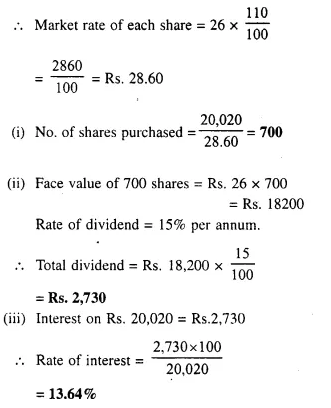Hope given Selina Concise Mathematics Class 10 ICSE Solutions Chapter 3 Shares and Dividend Ex 3B are helpful to complete your math homework.

If you have any doubts, please comment below. Learn Insta try to provide online math tutoring for you.